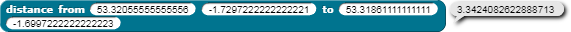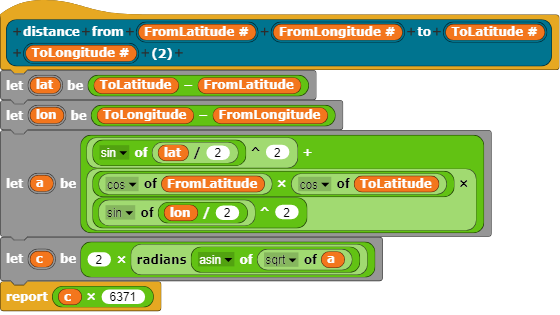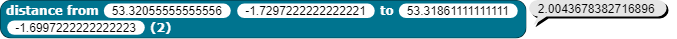# Help with haversine formula

Hello, I'm hoping someone can point out the issue in this distance block.

The distance between two spots on the planet is calculated using the haversine formula (longitude, latitude of the 2 spot)

You didn't post the code!

sorry !

@loucheman @bh
Probably this is the code.Example:There in a problem in the formula, i dont get the good result... with these parameters, the result should be 2.0043678382716137 Kilometers.

i got thisOh, thank you very much!

It seem perfect, i will test it.

Why the result isn't exactly the same? Is it because of the decimal data type?

It's because of floating point arithmetic, so yes.

the difference you're questioning at that precision is probably less than the thickness of paper!

The distance is in km i know, i'm just curious!

It's 75.9 picometers different. Compare that to 50 million to 100 million picometers as the thickness of a sheet of paper. So yes, it's smaller than the thickness of paper.

(Yes, I did the math there.)that's awesome!

This topic was automatically closed 30 days after the last reply. New replies are no longer allowed.

I've run into an issue with this node - it doesn't seem to be giving the correct result on my computer

I wonder if somone could check it out on theirs please?

I wasn't getting correct distances in a real project and even trying the values mentioned in the block iteself is giving the wrong answer

But I can't see any error in the JS -> Snap! conversion so its a bit strange

This is all a bit theoretical as the new map library loads a primitive that can be used instead

but it would be nice to know where the original is going wrongThere is no need to convert lat. long. to radians.
But the result of arcus sinus must be converted to radians, or the constant derived from the circumference of the Earth should be modified
$$6371 \approx \frac {40075} {2\pi}$$
to
$$113 \approx \frac {40075} {360\degree}$$.

#LOL